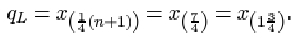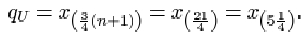Exploring data: Graphs and numerical summaries

This free course is available to start right now. Review the full course description and key learning outcomes and create an account and enrol if you want a free statement of participation.

Free course

5.6.2 Quartiles when the sample size is awkward

For the six ordered data items 1, 3, 3, 6, 7, 7, the lower quartile is given byIn other words, the lower quartile qL is given by the number three-quarters of the way between x (1)=1 and x (2)=3. The difference between x (1) and x (2) is 2, so

qL = x (1) + ¾(x (2)x (1)) = 1 + ¾ × 2 = 2.5.

The upper quartile is given bySo the upper quartile qu is the number one-quarter of the way between x (5)=7 and x (6)=7. This is just the number 7 itself.

Having defined the quartiles, it is straightforward to define the measure of dispersion based on them: the interquartile range is the difference between the quartiles.

The interquartile range

The interquartile range, which is a measure of the dispersion in a data set, is the difference qU−qL between the upper quartile qU and the lower quartile qL.

M248_1